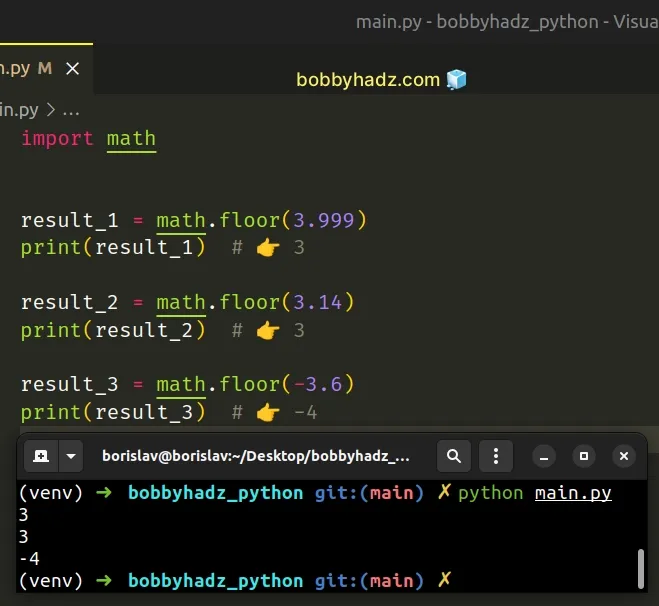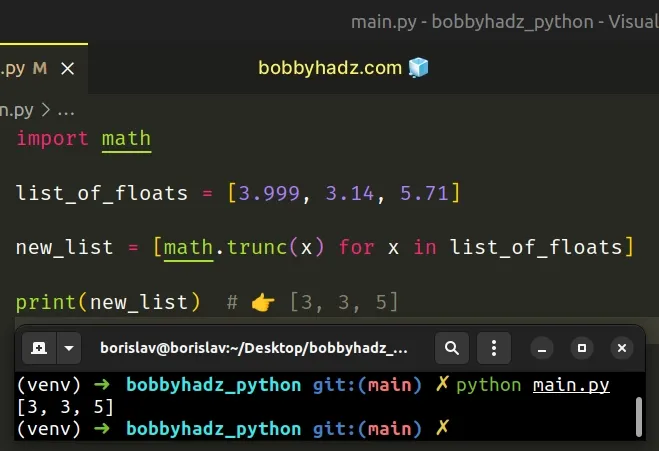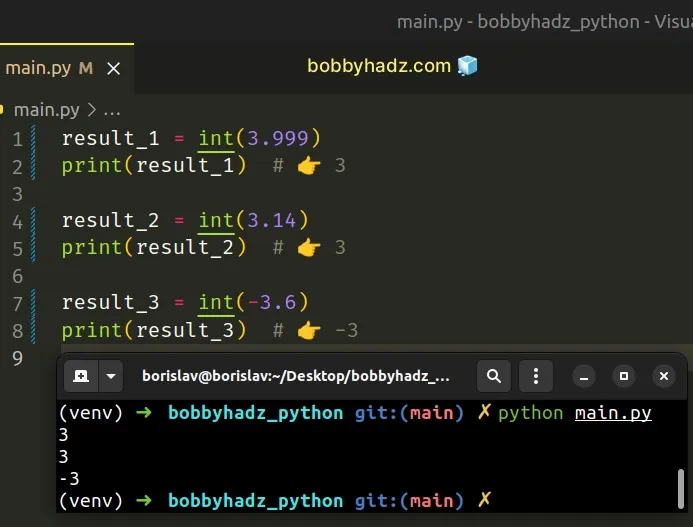# Remove the Decimal part from a Float in PythonLast updated: Feb 20, 2023
5 min## #Remove the decimal part from a Float using math.floor()

Use the `math.floor()` method to remove the decimal part from a float, e.g. `result = math.floor(3.999)`.

The `math.floor` method returns the largest integer less than or equal to the provided number.

main.py
```Copied!```import math

result_1 = math.floor(3.999)
print(result_1)  # 👉️ 3

result_2 = math.floor(3.14)
print(result_2)  # 👉️ 3

result_3 = math.floor(-3.6)
print(result_3)  # 👉️ -4
``````We used the `math.floor()` method to round a floating-point number down to the nearest integer.

main.py
```Copied!```import math

print(math.floor(6.99))  # 👉️ 6
print(math.floor(6.01))  # 👉️ 6
print(math.floor(-6.01))  # 👉️ -7
``````

The math.floor method returns the largest integer less than or equal to the provided number.

When passed a negative number, the `math.floor()` method rounds towards negative infinity.

This is different than the int() class, which rounds toward zero.

## #Remove the decimal part from a List of Floats

You can use a list comprehension if you need to remove the decimal part from a list of floats.

main.py
```Copied!```import math

list_of_floats = [3.999, 3.14, 5.71]

new_list = [math.trunc(x) for x in list_of_floats]

print(new_list) # 👉️ [3, 3, 5]
``````We used a list comprehension to iterate over the list and called the `math.trunc()` method with each float.

## #Remove the decimal part from a Float using int()

Alternatively, you can use the `int()` class.

main.py
```Copied!```result_1 = int(3.999)
print(result_1)  # 👉️ 3

result_2 = int(3.14)
print(result_2)  # 👉️ 3

result_3 = int(-3.6)
print(result_3)  # 👉️ -3
``````The `int()` class truncates floating-point numbers toward zero, so it will return an `int` that represents the number without the decimal places.

If you need to remove the decimal part from a list of floats, use a list comprehension.

main.py
```Copied!```list_of_floats = [3.999, 3.14, 5.71]

new_list = [int(x) for x in list_of_floats]

print(new_list)  # 👉️ [3, 3, 5]
``````

The int class returns an integer object constructed from the provided number or string argument.

If passed a floating-point number, the `int()` class truncates towards zero.
main.py
```Copied!```print(int(-11.1)) # 👉️ -11
print(int(-13.9)) # 👉️ -13
``````

Notice that there is a difference in how `math.floor()` and `int()` handle negative numbers:

• `math.floor()` rounds towards negative infinity
• `int()` truncates towards zero

## #Remove the decimal part from a Float using math.trunc()

If you need t round down, use the `math.trunc()` method.

main.py
```Copied!```import math

result_1 = math.trunc(3.999)
print(result_1)  # 👉️ 3

result_2 = math.trunc(3.14)
print(result_2)  # 👉️ 3

result_3 = math.trunc(-3.6)
print(result_3)  # 👉️ -3
``````

The math.trunc method takes a number, removes its fractional part and returns its integer part.

The `math.trunc()` method rounds towards zero.

main.py
```Copied!```import math

print(math.trunc(-9.1))  # 👉️ -9
print(math.trunc(-9.9))  # 👉️ -9
``````

This approach achieves the same result as passing the floating-point number to the `int()` class.

## #Remove the decimal part from a Float using math.ceil()

You can also use the `math.ceil()` method to remove the decimal part from a float by rounding up.

main.py
```Copied!```import math

result_1 = math.ceil(3.999)
print(result_1)  # 👉️ 4

result_2 = math.ceil(3.14)
print(result_2)  # 👉️ 4

result_3 = math.ceil(-3.6)
print(result_3)  # 👉️ -3
``````

The math.ceil method returns the smallest integer greater than or equal to the provided number.

## #Remove the decimal part from a Float using round()

You can also use the `round()` function to remove the decimal part from a float.

main.py
```Copied!```result_1 = round(3.999)
print(result_1)  # 👉️ 4

result_2 = round(3.14)
print(result_2)  # 👉️ 3

result_3 = round(-3.6)
print(result_3)  # 👉️ -4
``````

The round function takes the following 2 parameters:

NameDescription
`number`the number to round to `ndigits` precision after the decimal
`ndigits`the number of digits after the decimal, the number should have after the operation (optional)

The `round` function returns the number rounded to `ndigits` precision after the decimal point.

If `ndigits` is omitted, the function returns the nearest integer.

## #Remove the decimal part from a Float using split()

You can also use the `str.split()` method to remove the decimal part from a float.

main.py
```Copied!```a_float = 3.14

a_list = str(a_float).split('.')

print(a_list)

integer_part = int(a_list)
print(integer_part) # 👇️ 3
``````

The str.split() method splits the string into a list of substrings using a delimiter.

The method takes the following 2 parameters:

NameDescription
separatorSplit the string into substrings on each occurrence of the separator
maxsplitAt most `maxsplit` splits are done (optional)

We converted the float to a string and split the string on the period.

## #Format a float as an integer in Python

If you need to format a float as an integer, use a formatted string literal.

main.py
```Copied!```import math

my_float = 378.656789

# ✅ format float as int by removing fractional part (truncating)
result = f'{math.trunc(my_float)}'
print(result)  # 👉️ 378

# ----------------------------------------------

# ✅ format float as int with rounding
result = f'{my_float:.0f}'
print(result)  # 👉️ 379

# ----------------------------------------------

# ✅ format float as int by rounding up
result = f'{math.ceil(378.000001)}'
print(result)  # 👉️ 379
``````

We used a formatted string literal to format a float as an integer.

The first example uses the `math.trunc()` method in a formatted string literal to remove the float's fractional part.
main.py
```Copied!```import math

my_float = 378.656789

result = f'Result: {math.trunc(my_float)}'
print(result)  # 👉️ Result: 378
``````

The math.trunc method takes a number, removes its fractional part and returns its integer part.

The `math.trunc()` method rounds towards zero.

main.py
```Copied!```import math

print(math.trunc(3.468))  # 👉️ 3
print(math.trunc(-3.468))  # 👉️ -3
``````

Formatted string literals (f-strings) let us include expressions inside of a string by prefixing the string with `f`.

main.py
```Copied!```my_str = 'The number is:'
my_int = 123

result = f'{my_str} {my_int}'
print(result)  # 👉️ The number is: 123
``````

Make sure to wrap expressions in curly braces - `{expression}`.

Formatted string literals also enable us to use the format-specific mini-language in expression blocks.

main.py
```Copied!```my_float = 378.656789

result = f'{my_float:.0f}'
print(result)  # 👉️ 379
``````

The digit after the period is the number of decimal places the number should have.

Notice that f-strings format with rounding.

You can use the `math.ceil()` method to round up when formatting the float as an integer.

main.py
```Copied!```import math

result = f'{math.ceil(378.000001)}'
print(result)  # 👉️ 379
``````

The math.ceil method returns the smallest integer greater than or equal to the provided number.

If you need to round down, use the `math.floor()` method.

main.py
```Copied!```import math

result = f'{math.floor(378.9999999)}'
print(result)  # 👉️ 378
``````

The math.floor method returns the largest integer less than or equal to the provided number.